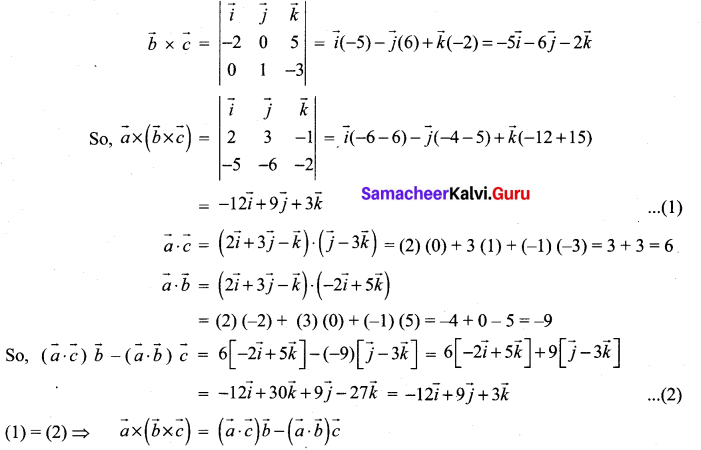# Samacheer Kalvi 12th Maths Solutions Chapter 6 Applications of Vector Algebra Ex 6.3

## Tamilnadu Samacheer Kalvi 12th Maths Solutions Chapter 6 Applications of Vector Algebra Ex 6.3

Question 1.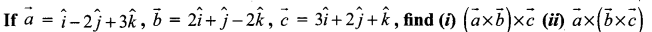Solution: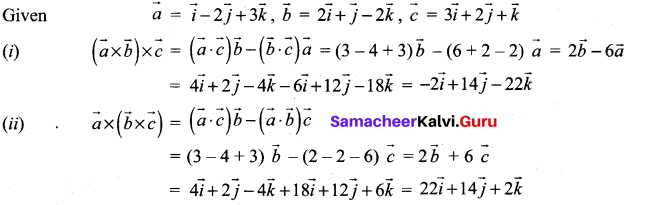Question 2.
For any vector $$\vec{a}$$, prove that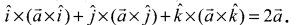Solution: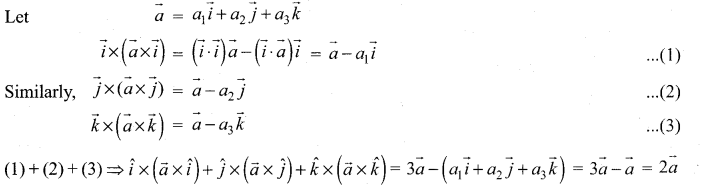Question 3.
Prove that $$[\vec{a}-\vec{b}, \vec{b}-\vec{c}, \vec{c}-\vec{a}]$$ = 0
Solution: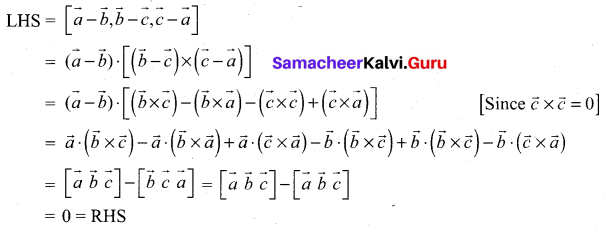Question 4.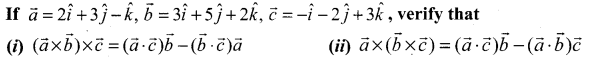Solution: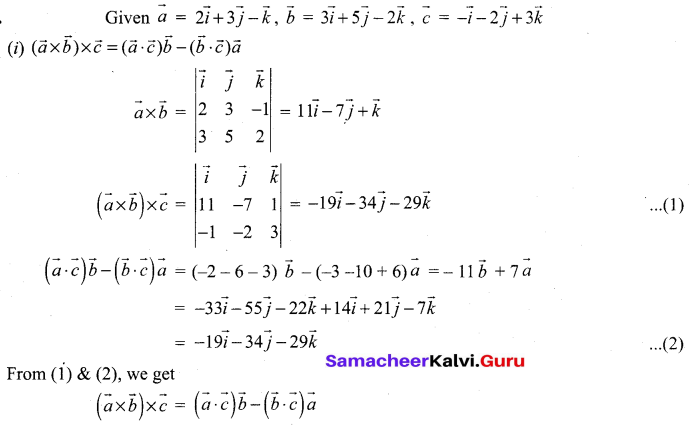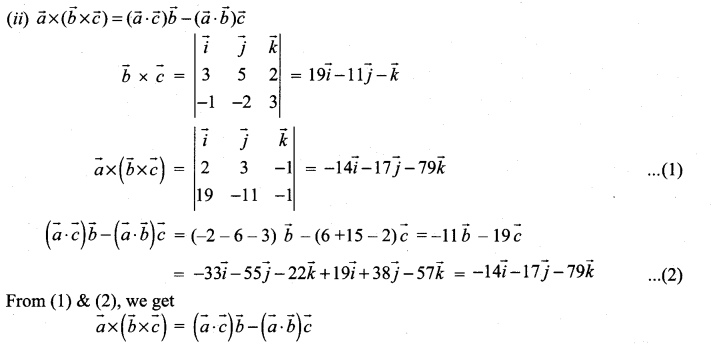Question 5.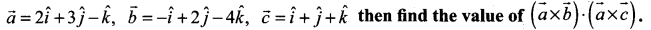Solution: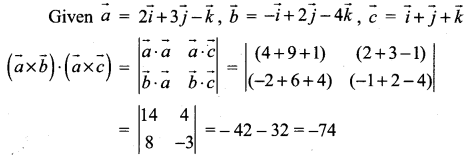Question 6.
If $$\vec{a}, \vec{b}, \vec{c}, \vec{d}$$ are coplanar vectors, show that $$(\vec{a} \times \vec{b}) \times(\vec{c} \times \vec{d})=\overrightarrow{0}$$
Solution: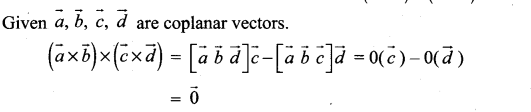Question 7.
If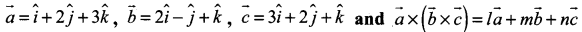, find the values of l, m, n
Solution: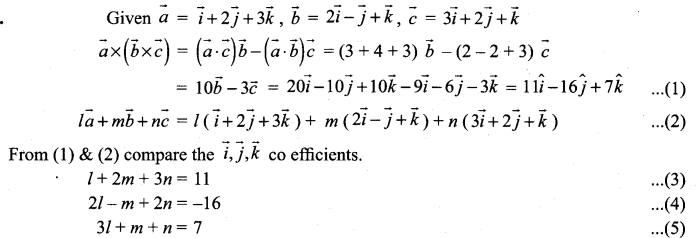On solving (3) & (4)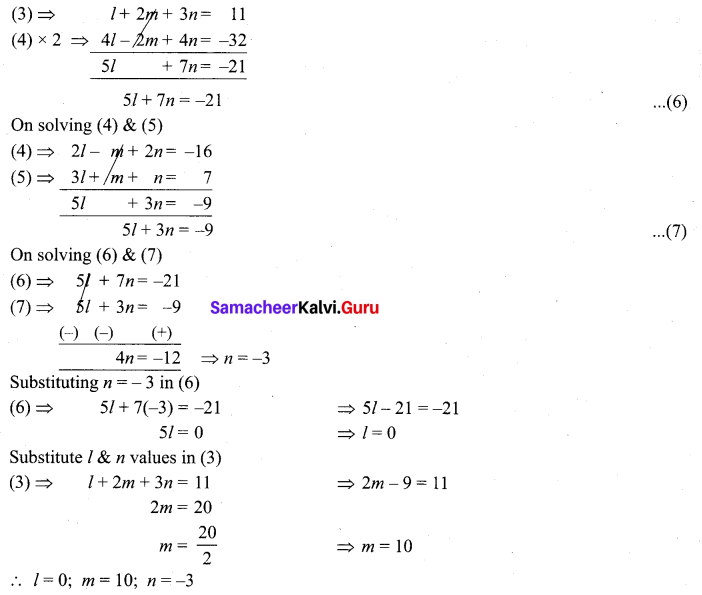Question 8.
If $$\hat{a}, \hat{b}, \hat{c}$$ are three unit vectors such that $$\hat{b} \text { and } \hat{c}$$ are non-parallel and $$\hat{a} \times(\hat{b} \times \hat{c})=\frac{1}{2} \hat{b}$$, find the angle between $$\hat{a}$$ and $$\hat{c}$$.
Solution: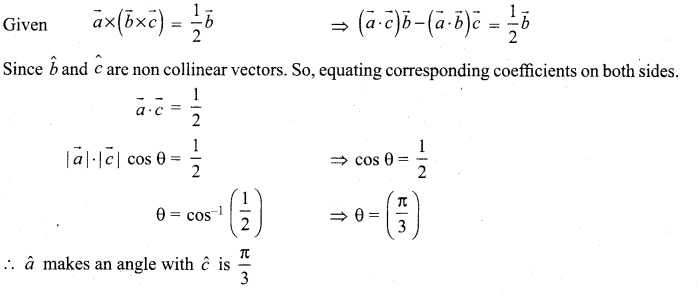### Samacheer Kalvi 12th Maths Solutions Chapter 6 Applications of Vector Algebra Ex 6.3 Additional Problems

Question 1.
If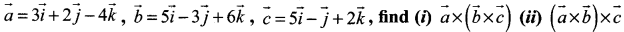and show that they are not equal.
Solution: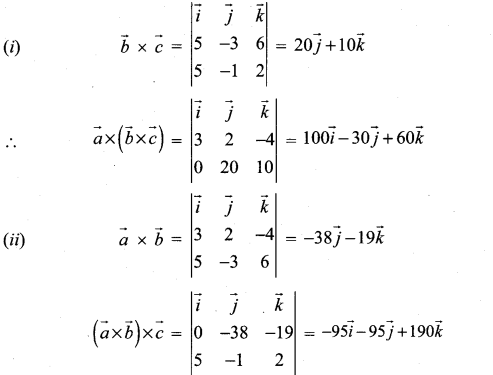Question 2.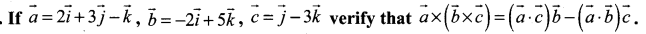Solution: